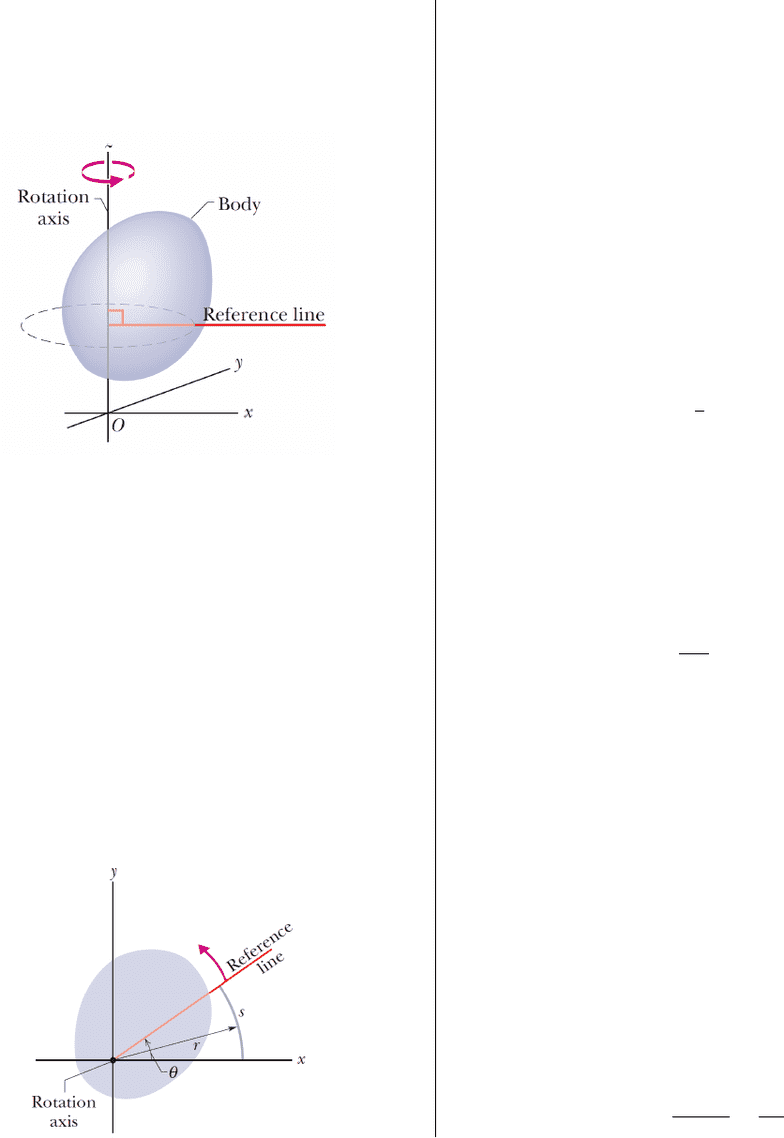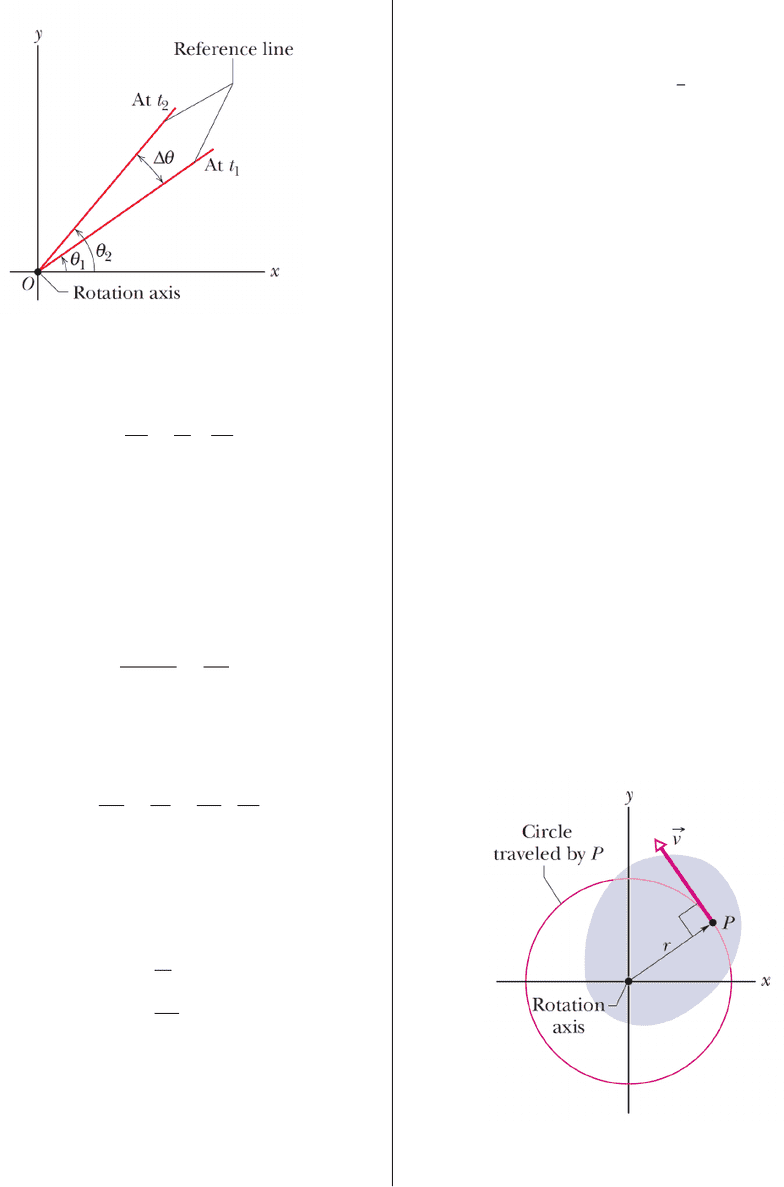Textbook Notes (270,000)
CA (160,000)
UTSG (10,000)
Physics (80)
all (30)
Study Guide

# Textbook Guide Physics: Kinematics, Moment Of Inertia, Ob River

Department
Physics
Course Code
PHY131H1
Professor
all

This preview shows pages 1-3. to view the full 10 pages of the document.Rotation
1 Rotational Variables
Rotation correspond to the motion of an object about a
given axis.
The proper study of this type of motion requires several
elements:
Deﬁne a good reference system. In the ﬁgure, zaxis
coincides with the rotation axis.
Deﬁne angular variables that simplify the math for
describing the position of the object respect the ref-
erence system.
Def. 1 (Rigid Body).A rigid body is a particle system where
the distances between all of them remain ﬁxed at all times. In
other words, the body does not suffer deformations.
A rigid body is sometimes called also solid.
Why we concentrate on the study of rigid bodies? Because
we can study the motion of the complete body paying at-
tention to a single point of the object, and then refer the
rest of the body to that point.
A very useful reference system selection is one where one
of the axis coincides with the rotation axis.
On that case, we can describe the position of each part of
the object using the angle between a given part of the ob-
ject and an arbitrary axis x.
Def. 2 (Angular Position).The angular position is the angle
of the reference line relative to a ﬁxed direction (xaxis), which
we take as the zero angular position.
The angular position is denoted by the letter θand its measured
in the counterclockwise direction.
θ=s
where sis the arc length deﬁned by the reference point and ris
the distance from this point to the rotation axis, which coincides
with the origin of the reference system.
or revolutions. A complete revolution describes an arc of
s= 2πr so the angle is
θ=2πr
r= 2π.
Def. 3 (Angular Displacement).The angular displacement
correspond to the angle difference measured at two different
times. It is represented by θand its value is
θ=θ2θ1.
Obs. 1. Angle displacements can be positive or negative de-
pending on the direction of the rotation.
Positive: the body rotates counterclockwise.
Negative: the body rotates clockwise.
Def. 4 (Angular Velocity).Let θ1be the angular position at
t=t1and θ2be the angular position at t=t2.
The average angular velocity on the interval [t1, t2]is
ωavg =θ2θ1
t2t1
=θ
t.
1

Only pages 1-3 are available for preview. Some parts have been intentionally blurred.Def. 5 (Instantaneous Angular Velocity ω).The instanta-
neous angular velocity, denoted by ω, is the limit of the the av-
erage angular velocity when t0:
ω= lim
t0
θ
t
s.(2)
The magnitude of the |ω|is the angular speed.
The angular acceleration corresponds to the variation of
angular velocity for a rotating body.
Def. 6 (Average Angular Acceleration).Let ω1and ω2be the
angular velocities at t1and t2, respectively. Then, the average
angular velocity αavg is:
αavg =ω2ω1
t2t1
=ω
t.
Def. 7 (Instantaneous Angular Acceleration).The instan-
taneous angular acceleration, denoted by α, is the limit average
angular acceleration when t0, i.e.,
α= lim
t0
ω
t=
dt =d2θ
s2.(3)
Obs. 2 (Dot Notation for Time Derivatives).In mechanics
it is very common to use an special notation for time derivatives.
This is the “dot notation” for time differentiation. In other
words, we write
˙
θ
dt
¨
θd2θ
dt2
2 Rotation with Constant Angular Ac-
celeration
This is the ﬁrst type of rotational motion we are going to
study.
In this case, αis constant, so we can integrate (3) to obtain:
ω(t) = ω0+αt (4a)
θ(t) = θ0+ω0t+1
2αt2(4b)
ω2(t) = ω2+ 2αθ(t)θ0(4c)
where:
θ0is the angular displacement at t= 0.
ω0is the angular velocity at t= 0.
These terms are known as initial conditions.
3 Relating Linear and Angular Vari-
ables
Let’s remember that the description of the motion of a par-
ticle respect a ﬁxed reference system is done using three
variables:
~r or position
~v or velocity
~a or acceleration
But in this chapter we have introduced three additional
variables to describe rotation:
θor angular position
ωor angular velocity
αor angular acceleration
Let’s consider the case of a particle Pmoving along a circle
arc of radius r.
2

Only pages 1-3 are available for preview. Some parts have been intentionally blurred.We want to express ~r,~v, and ~a in terms of r,θ,ωand α.
One of the issues with making these computations is the
fact that although the distance from Pto the origin is ﬁxed,
the direction of the vector ~r changes at each instant.
Therefore, this direction change implies that the velocity
of Pis not zero.
How do we know this?
3.1 Detour: Unitary Vectors
The deﬁnition of a coordinate system x, y, z such as the
one depicted in the ﬁgure induces the existence of a base
of three unitary vectors ˆ
i,ˆ
j, and ˆ
kthat are parallel to the
axis x,y, and zrespectively.
These vectors are ﬁxed in time and they have the following
properties:
ˆ
i·ˆ
i=ˆ
j·ˆ
j=ˆ
k·ˆ
k= 1
ˆ
i·ˆ
j=ˆ
i·ˆ
k=ˆ
j·ˆ
k= 0.
In other words, the three vectors are perpendicular - hence
the dot product is zero between them - and there magni-
tude is 1.
3.2 Position of P
We can express the position of Pin terms of the unitary
vectors ˆ
iand ˆ
jpreviously deﬁned. Thus,
~r =rcos(θ)ˆ
i+rsin(θ)ˆ
j.
Note that we can deﬁne a unitary vector ˆralong ~r such
that |ˆr|= 1 if and only if
ˆr= cos(θ)ˆ
i+ sin(θ)ˆ
j.
Hence, the position of Pcan be expressed compactly by
~r =rˆr. (5)
3.3 Velocity of P
The velocity vector is
~v =d~r
dt
=drˆr
dt
=dr
dt ˆr+rdˆr
dt
Since ˆr= cos(θ)ˆ
i+ sin(θ)ˆ
jdepends on time indirectly
through θ, we cannot dismiss this term.
In fact:
dˆr
dt =d
dtcos(θ)ˆ
i+ sin(θ)ˆ
j
=sin(θ)
dtˆ
i+ cos(θ)
dt ˆ
j
=
dt sin(θ)ˆ
i+ cos(θ)ˆ
j.
We can deﬁne a second unitary vector ˆ
θby
ˆ
θ sin(θ)ˆ
i+ cos(θ)ˆ
j(6)
This vector is perpendicular to ˆrsince ˆr·ˆ
θ= 0. In addition,
|ˆ
θ|= 1 as well.
Therefore, the velocity of Pis
~v =dr
dt ˆr+r
dt ˆ
θ. (7)
Since Premains at constant distance from the origin, then
dr/dt = 0 and
~v =rωˆ
θ. (8)
Note that the speed of Pis quite simple to compute since
it’s the term that’s next to ˆ
θ:
v=|~v|=rω.
3.4 Acceleration of P
The acceleration of Pcan be computed taking a time
derivative of ~v.
~a =d~v
dt
=d
dtrωˆ
θ
=r
dt ˆ
θ+rω dˆ
θ
dt
The second term can be computed by determining dˆ
θ/dt.
dˆ
θ
dt =d
dtsin(θ)ˆ
i+ cos(θ)ˆ
j
=cos(θ)
dtˆ
isin(θ)
dt ˆ
j
=
dt ˆr
=ωˆr
Therefore,
~a =rω2ˆr+rαˆ
θ(9)
The acceleration of Pit’s due to two effects:
The change on direction of P; this is the centripetal
acceleration of magnitude rω2.
The angular acceleration αwhich induces a tangential
acceleration rα.
3
###### You're Reading a Preview

Unlock to view full version

Only pages 1-3 are available for preview. Some parts have been intentionally blurred.###### You're Reading a Preview

Unlock to view full version